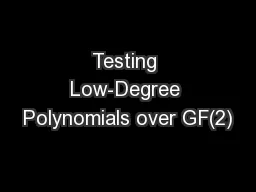# Testing Low-Degree Polynomials over GF(2)Embed code:

## Testing Low-Degree Polynomials over GF(2)

Download Presentation - The PPT/PDF document "Testing Low-Degree Polynomials over GF(2..." is the property of its rightful owner. Permission is granted to download and print the materials on this web site for personal, non-commercial use only, and to display it on your personal computer provided you do not modify the materials and that you retain all copyright notices contained in the materials. By downloading content from our website, you accept the terms of this agreement.

### Presentations text content in Testing Low-Degree Polynomials over GF(2)

Slide1

Testing Low-Degree Polynomials over GF(2)

Noga

Alon

Simon Litsyn

Michael Krivelevich

Tali Kaufman

Dana Ron

Danny

Vainstein

Slide2

Definitions

Slide3

Definitions

Let

P

k

be all polynomials over {0,1}

n

with degree at most k without a free term (over GF(2)).

Slide4

Definitions

Let

Pk be all polynomials over {0,1}n with degree at most k without a free term (over GF(2)).

Slide5

Definitions

Let

Pk be all polynomials over {0,1}n with degree at most k without a free term (over GF(2)).

Slide6

Definitions

Let

Pk be all polynomials over {0,1}n with degree at most k without a free term (over GF(2)).

Slide7

Definitions

For any two functions :

The

symmetric difference is:

The

relative distance

is:

Slide8

Definitions

For any two functions :

The

symmetric difference is:

The

relative distance

is:

For a function

f

and a family of functions G, we say that f is -far from G, for some if for every ,

Slide9

Definitions

Slide10

Definitions

Slide11

Definitions

Slide12

Definitions

Slide13

Definitions

Slide14

Definitions

Slide15

Definitions

Slide16

Definitions

Slide17

Characterization Theorem

Slide18

Characterization Theorem

Slide19

Characterization Theorem

Slide20

Slide21

Slide22

Slide23

Slide24

Slide25

Slide26

Slide27

Slide28

Slide29

Slide30

Slide31

Slide32

Slide33

Slide34

Slide35

Slide36

Slide37

Slide38

Slide39

Slide40

Slide41

Slide42

Slide43

Slide44

Slide45

Slide46

Slide47

Characterization Theorem- Reminder

Slide48

The Algorithm

Slide49

The Algorithm

Slide50

The Algorithm

Slide51

The Algorithm – cont.

Slide52

The Algorithm – cont.

Slide53

The Algorithm – cont.

Slide54

Definitions

Slide55

Definitions

Slide56

Definitions

Slide57

Lemmas

Slide58

Lemmas

Slide59

Lemmas

Slide60

Proof of Correctness

Slide61

Proof of Correctness

Slide62

Proof of Correctness

Slide63

Proof of Correctness

Slide64

Proof of Correctness

Slide65

Proof of Correctness

Slide66

Proof of Correctness

Slide67

Proof of Correctness

Slide68

Proof of Correctness

Slide69

Proof of Correctness

Slide70

Proof of Correctness

Slide71

Proof of Correctness

Slide72

Questions?

Slide73

Slide74

Slide75

Slide76